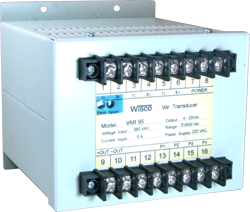# VAR95: Var Transducer Vietnam

 Voltage Input 110 VAC, 220 VAC, 380 VAC - Current Input 1 A, 5 A - Analog Output 4-20 mA, 0-5 VDC

The Var Transducers VAR95  are used to convert single or three phase unbalance load reactive power into a proportional load independent DC. output. Based fully electronic Time-Division Multiplication measuring principle, the reactive power of a network with any power factor range are linearly produced by Var Transducers output.

Calibration var of rated output

Connection V=110V V=220V V=380V

 I=1A I=5A
 I=1A I=5A
 I=1A I=5A
Single Phase
 ±100var ±500var
 ±200var ±1000var
 - -
3phase,3wire
 ±200var ±1000var
 ±400var ±2000var
 ±600var ±3000var
3phase,4wire
 ±200var ±1000var
 ±400var ±2000var
 ±600var ±3000var

Note. V= Line voltage

Equation to calculated measuring range

Measuring range = Calibration var x PT ratio x CT ratio

Example 3phase,3wire, PT 220V/110V, CT 250/5A, Calibration var = 1000W

Measuring range = 1000 x (220/110) x (250/5) = 100 Kvar.